# GMAT Math : Data Interpretation

## Example Questions

← Previous 1

### Example Question #1 : Dsq: Calculating Probability

A fair coin is tossed a given number of times. What is the probability that three or more of the tosses will come up heads?

1) The number of times the coin is tossed is four.

2) The previous day, the coin was tossed ten times, and heads came up eight times.

BOTH statements TOGETHER are not sufficient to answer the question.

BOTH statements TOGETHER are sufficient to answer the question, but NEITHER statement ALONE is sufficient to answer the question.

Statement 1 ALONE is sufficient to answer the question, but Statement 2 ALONE is not sufficient to answer the question.

Statement 2 ALONE is sufficient to answer the question, but Statement 1 ALONE is not sufficient to answer the question.

EITHER statement ALONE is sufficient to answer the question.

Statement 1 ALONE is sufficient to answer the question, but Statement 2 ALONE is not sufficient to answer the question.

Explanation:

This is a binomial probability experiment.

The probability that a fair coin will come up heads in any single toss isIf the coin is tossed four times, then the probability that heads will appear three times is: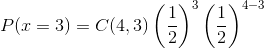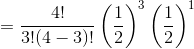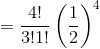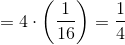The probability that heads will appear four times is: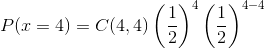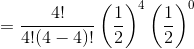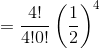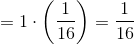So the probability that heads will appear at least three times is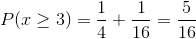The second statement is irrelevant; the result of a previous trial has no influence on that of the current trial. It has already been established in the problem that the coin is fair.

The answer is that Statement 1 alone is sufficient to answer the question, but not Statement 2.

### Example Question #61 : Data Sufficiency Questions

A monster is holding a brown paper bag filled with cookies. Inside the bag there are two types of cookies, chocolate chip and oatmeal raisin. If the monster pulls one cookie out at random, what is the probability that he pulls out a chocolate chip cookie.

1. There are a total of 24 cookies in the bag.

2. There are 8 more chocolate chip cookies in the bag than oatmeal raisin cookies.

BOTH statements TOGETHER are sufficient, but NEITHER statement ALONE is sufficient.

Statements (1) and (2) TOGETHER are NOT sufficient.

Statement (1) ALONE is sufficient, but statement (2) alone is not sufficient.

EACH statement ALONE is sufficient.

Statement (2) ALONE is sufficient, but statement (1) alone is not sufficient.

BOTH statements TOGETHER are sufficient, but NEITHER statement ALONE is sufficient.

Explanation:

We need both statements to get the answer.

Statement 1 alone is not enough. Having 24 total cookies does not tell us the proportions of each type of cookie. We could have 1 chocolate chip cookie and 23 oatmeal raisin, or vice versa.

Statement 2 alone is not sufficient either. Knowing that there are 8 more chocolate chip cookies than oatmeal raisin without knowing the total amount in the bag does not help. It could be 1 oatmeal raisin cookie and 9 chocolate chip, or it could be 100 oatmeal raisin cookies and 108 chocolate chip cookies (although that is a pretty big bag!)

Only by having both statements together can we find the right proportion. If we letbe the number of chocolate chip cookies, andbe the number of oatmeal raisin, we can set two equations and find the right ratio. We know that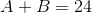from statement 1, andfrom statement 2.

Substitutinginto the first equation we get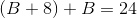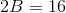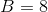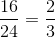or 66.67% chance that the monster pulls out a chocolate chip cookie.

### Example Question #1 : Dsq: Calculating Probability

Choose the answer that best describes sufficient data to solve the problem.

A student body is polled regarding their favorite campus food.  Of those who answered "Pizza" half were male and half were female.  Pizza received 24 votes from men.

I.  Pizza received the votes of 1 out of every 5 men and 3 out of every 10 women.

II. The student population was found to be 60% male and 40% female

How many students altogether were polled?

Either statement alone is sufficient to solve the problem.

Statement I is sufficient to solve the problem, but Statement II alone is not.

Neither statement is sufficient to solve the problem (additional information is needed).

Statement II is sufficient to solve the problem, but Statement I alone is not.

Both statements together are sufficient to solve the problem.

Statement I is sufficient to solve the problem, but Statement II alone is not.

Explanation:

Pizza received 24 votes from men. Since half of those who responded pizza were women, there must be 24 votes from women.

If 1 out of 5 men voted for pizza, this means we have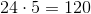men.  If 3 out of 10 women voted for pizza, we have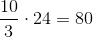women for a total of 200 people polled.

If our population is instead given as 60% male and 40% female, we still have 24 men and 24 women that voted for pizza, but while we can determine the ratio of people who voted, we cannot get a precise number.  For example, while 80 women and 120 men works, we could also have 160 women and 240 men as our total poll (with 1 out of 10 men and 3 out of 20 women voting pizza).

Thus the first condition is sufficient while the second is not.

### Example Question #1 : Probability

Find the probability.

Statement 1): Given that the lastflips of a coin were heads, the next coin flip is heads.

Statement 2): Given that the lastdays were sunny skies, the next day is sunny.

BOTH statements taken TOGETHER are sufficient to answer the question, but neither statement ALONE is sufficient.

Statement 1) ALONE is sufficient, but Statement 2) ALONE is not sufficient to answer the question.

Statement 2) ALONE is sufficient, but Statement 1) ALONE is not sufficient to answer the question.

BOTH statements TOGETHER are NOT sufficient, and additional data is needed to answer the question.

EACH statement ALONE is sufficient.

Statement 1) ALONE is sufficient, but Statement 2) ALONE is not sufficient to answer the question.

Explanation:

This question deals with conditional probability.

Statement 1) consists of independent events. The past trials will not affect the future trials of the coin.  The odds of flipping a coin and land on heads will be.

Statement 2) also consists of independent events. However, given that the previous 10 days were sunny does not necessarily indicate that the next day will guarantee that it will be a sunny day.  The probability is unknown because the statement does not give any information about the percentage of a sunny sky on any particular day.

### Example Question #64 : Word Problems

Jenkins has a box of 36 guitar picks. Each pick is one of three colors: blue, black, or orange.

I) There are 14 more orange picks than blue picks.

II) There are 12 black picks.

What are the odds of randomly selecting a black pick, followed by a blue pick, followed by an orange pick?

Statement II is sufficient to answer the question, but Statement I is not sufficient to answer the question.

Statement I is sufficient to answer the question, but Statement II is not sufficient to answer the question.

Both statements are needed to answer the question.

Either statement is sufficient to answer the question.

Both statements are needed to answer the question.

Explanation:

To find the probability of several events, we need to find the probability of each event separately. First, we need to know how many there are of each color pick.

Statement I relates the number of orange and blue picks.

Statement II tells us the number of black picks.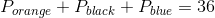From Statement I: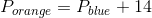From Statement II: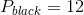Using Statement I, Statement II, and the given info, we can substitute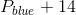from Statement I into Statement II for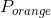and get the following: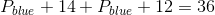We can then solve for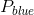: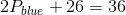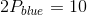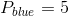Since we are told in Statement I that there are 14 more blue picks than orange picks, we can then find the number of orange picks: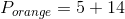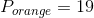So, to find the odds of multiple events, multiply the probability of each event together: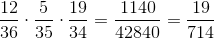### Example Question #1 : Dsq: Understanding Diagrams

Below is a menu of the drinks served at Moonbucks Coffee: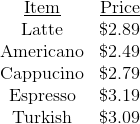Today and today only, there is a special - buy two espressos and get any third drink free. There are no other specials.

Ralph and Janet are each ordering three beverages from this menu. Who, if either, will pay more?

Statement 1: Two of Ralph's drinks are espressos.

Statement 2: Two of Janet's drinks are cappucinos.

Statement 1 ALONE is sufficient to answer the question, but Statement 2 ALONE is NOT sufficient to answer the question.

Statement 2 ALONE is sufficient to answer the question, but Statement 1 ALONE is NOT sufficient to answer the question.

BOTH statements TOGETHER are sufficient to answer the question, but NEITHER statement ALONE is sufficient to answer the question.

BOTH statements TOGETHER are insufficient to answer the question.

EITHER statement ALONE is sufficient to answer the question.

BOTH statements TOGETHER are sufficient to answer the question, but NEITHER statement ALONE is sufficient to answer the question.

Explanation:

Each statement alone gives information about only one order, so at the very least, both statements will be necessary.

From Statement 1, it is known that Ralph will pay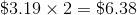for his drinks, since he will only pay for the two espressos.

From Statement 2, it cannot be determined exactly what Janet will pay. However, she will pay for all three drinks, two of which are cappucinos; the least she will spend is the price of two cappucinos and an Americano, which is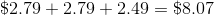.

From both statements together, it is definite that Janet will pay more.

### Example Question #61 : Data Sufficiency Questions

Below is a menu of the drinks served at Moonbucks Coffee:Patty and Mickey each order three beverages from the menu. Who, if either, will pay more (disregard tax)?

Statement 1: All three of Patty's drinks are Turkish coffees.

Statement 2: One of Mickey's drinks is an Americano.

BOTH statements TOGETHER are insufficient to answer the question.

Statement 2 ALONE is sufficient to answer the question, but Statement 1 ALONE is NOT sufficient to answer the question.

BOTH statements TOGETHER are sufficient to answer the question, but NEITHER statement ALONE is sufficient to answer the question.

EITHER statement ALONE is sufficient to answer the question.

Statement 1 ALONE is sufficient to answer the question, but Statement 2 ALONE is NOT sufficient to answer the question.

BOTH statements TOGETHER are sufficient to answer the question, but NEITHER statement ALONE is sufficient to answer the question.

Explanation:

From Statement 1 alone, you know that Patty spent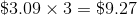. It is possible, however, that Mickey spent more (for three espressos, for example), just as much (on three Turkish coffees), or less (for three cappucinos, for example). Statement 2 alone gives you even more scant information, since it only tells you one beverage Mickey bought.

Suppose you know both statements. Then you know Patty spent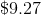. You do not know how much Mickey spent, but the most he could have spent was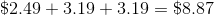(for an Americano and two esperessos). Therefore, the two statements together are necessary and sufficient to answer the question.

### Example Question #61 : Word Problems

Below are the results of the initial election for senior class president.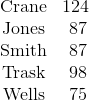No candidate won a majority, so Crane and Trask faced each other in a runoff. Every student in the senior class voted in both the initial election and the runoff. Every student who voted for Crane in the initial election voted for Crane in the runoff, and every student who voted for Trask in the initial election voted for Trask in the runoff.

Statement 1: All of the students who voted for Jones in the initial election voted for Crane.

Statement 2: Two-thirds of the students who voted for Wells in the initial election voted for Trask.

Statement 2 ALONE is sufficient to answer the question, but Statement 1 ALONE is NOT sufficient to answer the question.

BOTH statements TOGETHER are insufficient to answer the question.

Statement 1 ALONE is sufficient to answer the question, but Statement 2 ALONE is NOT sufficient to answer the question.

BOTH statements TOGETHER are sufficient to answer the question, but NEITHER statement ALONE is sufficient to answer the question.

EITHER statement ALONE is sufficient to answer the question.

BOTH statements TOGETHER are sufficient to answer the question, but NEITHER statement ALONE is sufficient to answer the question.

Explanation: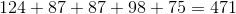students voted, so 236 votes were necessary to win a simple majority.

From Statement 1 alone it can be determined that Crane won at least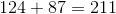votes (his own initial votes plus all of the votes that previously went to Jones), and Trask won at least 98 (his own initial votes).

From Statement 2 alone, it can be determined that Crane won at least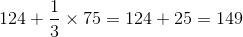(his own initial votes plus one-third of the votes that previously went to Wells), and Trask won at least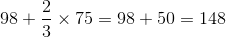votes (his own initial votes plus two-thirds of the votes that previously went to Wells).

Neither statement alone will prove a majority vote. From Statement 1 and 2 together, however, it is known that Crane won at least his own initial votes, all of Jones' votes, and one-third of Wells' votes - a minimum of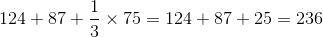votes. Therefore Crane is the winner, and both statements are needed to prove this.

### Example Question #62 : Word Problems

The table below gives the population of the city of Renfrow for each of six census years.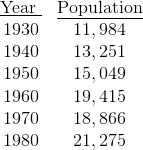Did Renfrow have a larger population in 1945 or 1955?

Statement 1: The population rose every year from 1940 to 1950.

Statement 2: The population rose every year from 1950 to 1960.

Statement 1 ALONE is sufficient to answer the question, but Statement 2 ALONE is NOT sufficient to answer the question.

BOTH statements TOGETHER are insufficient to answer the question.

Statement 2 ALONE is sufficient to answer the question, but Statement 1 ALONE is NOT sufficient to answer the question.

EITHER statement ALONE is sufficient to answer the question.

BOTH statements TOGETHER are sufficient to answer the question, but NEITHER statement ALONE is sufficient to answer the question.

BOTH statements TOGETHER are sufficient to answer the question, but NEITHER statement ALONE is sufficient to answer the question.

Explanation:

Statement 1 alone tells us that the population of Renfrow in 1945 was between 13,251 and 15,049, but says nothing about the population in 1955.

Similarly, Statement 2 alone tells us that the population in 1955 was between 15,049 and 19,415, but says nothing about the population in 1945.

The two statements together, however, tell us that the population rose every year from 1940 to 1960, so the population in 1955 had to have been greater than the population in 1945.

### Example Question #1 : Dsq: Understanding Diagrams

The table below gives the population of the city of Renfrow for each of six census years.Did Renfrow have a greater population in 1965 or 1975?

Statement 1: The population of Renfrow decreased every year from 1960 to 1970.

Statement 2: The population of Renfrow increased every year from 1970 to 1980.

BOTH statements TOGETHER are insufficient to answer the question.

BOTH statements TOGETHER are sufficient to answer the question, but NEITHER statement ALONE is sufficient to answer the question.

Statement 1 ALONE is sufficient to answer the question, but Statement 2 ALONE is NOT sufficient to answer the question.

Statement 2 ALONE is sufficient to answer the question, but Statement 1 ALONE is NOT sufficient to answer the question.

EITHER statement ALONE is sufficient to answer the question.